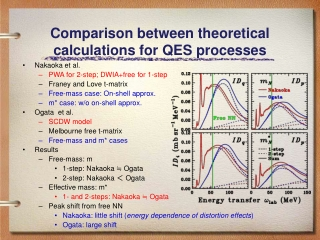DownloadDownload PresentationComparison between theoretical calculations for QES processes

# Comparison between theoretical calculations for QES processes

Download Presentation## Comparison between theoretical calculations for QES processes

- - - - - - - - - - - - - - - - - - - - - - - - - - - E N D - - - - - - - - - - - - - - - - - - - - - - - - - - -
##### Presentation Transcript

1. Comparison between theoretical calculations for QES processes • Nakaoka et al. • PWA for 2-step; DWIA+free for 1-step • Franey and Love t-matrix • Free-mass case: On-shell approx. • m* case: w/o on-shell approx. • Ogata et al. • SCDW model • Melbourne free t-matrix • Free-mass and m* cases • Results • Free-mass: m • 1-step: Nakaoka ≒ Ogata • 2-step: Nakaoka ＜ Ogata • Effective mass: m* • 1- and 2-steps: Nakaoka ≒ Ogata • Peak shift from free NN • Nakaoka: little shift (energy dependence of distortion effects) • Ogata: large shift

2. Peak shift mechanism andbinding-energy effect for QES • Energy transfer w for QES at q • Binding energy EB does not contribute to the peak shift • Peak shift depends on single particle potentials V and the effective mass m* • (p,n):Shift = 20-30 MeV • (p,p’):Shift = 0-10 MeV Peak Shift Width NN Peak

3. Peak shift mechanism andQ-value effect for QES • 12C(p,n) case • 40Ca(p,n) case • QES peak shift:Δω～20 MeV for 12C(p,n) and 40Ca(p,n) • Δω should depend on ΔV • ΔＶ　←→　Q　⇒　Δω　←→　Q QES peak should not (directly)depend on Q-value × ×

4. QES peak shift for light nuclei • 2H, 3He(p,n) • Q-values • 2H: -2.2 MeV (Break-up) • 3He: -1.8 MeV • Almost no shift • m* and DV effects:× • 4He(p,n) • Q-value: -23.7 MeV • Peak shift: ～10 MeV < Q-value • m* and DV effects ? Free NN ～10 MeV D.L Prout et al., Phys. Rev. C 65, 034611 (2002)

5. QES peak shift for C isotopes • 12C, 13C(p,n) • m* and distortion effects would be same • Q-values • 12C: -18.1 MeV • 13C: -3.0 MeV • Single particle potential • 12C: DV=22.8 MeV • 13C: DV= 0.5 MeV • Peak shift • 12C: Dw=25±3 MeV • 13C: Dw=14±7 MeV • Results • Peak-shift difference　～　Q-value difference • Peak-shift difference < DV difference • DV difference for C isotopes is physically appropriate? Free NN 25 MeV 14 MeV H. Otsu, Doctoral Dissertation Univ. of Tokyo (1997)

6. Distortion, m*, and correlation effects for QES peak shift • PWIA/DWIA calculations • PW vs. DW • m (free mass) vs. m* (effective mass) • Free response (0th)vs. RPA response • Contribution to peak shift • PW+m+Free response(0th)=Free NN • Distortion: +8 MeV • m*(effective mass): +6 MeV • RPA (correlation): +6 MeV • Total: +20 MeV